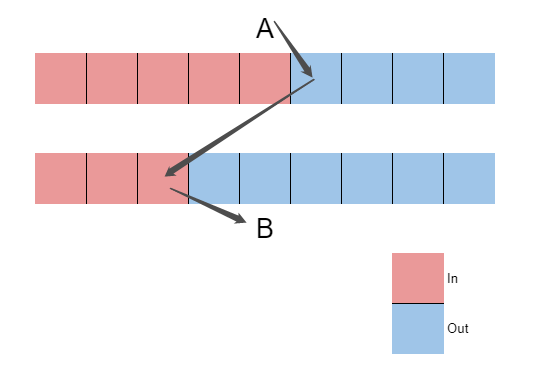## Solution:

Let me talk about the conclusion first.

For all pairs of points, we sort it from largest to smallest. Query the reachability of the points with higher in-degree to lower in-degree in these pairs in turn. If reachable, then these two point is the answer.

It is obvious after looking at the picture below:A have more in-degree point than B. And reachable to B.

We can found that: At least one of the out-degree points of $B$ must be the in-degree of $A$. So A point with a larger in-degree can reach a smaller point, and it must be a circle.

For two points with same in-degree. It's same, because it will take a in-degree point from $A$, and take a out-degree from $B$.

## Code:蜀ICP备19018968号